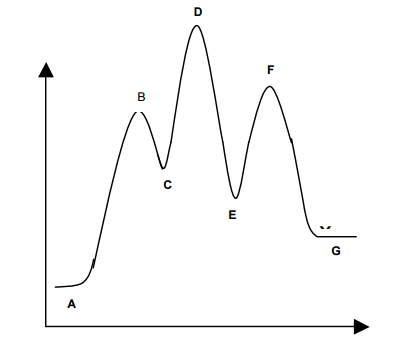# Problem: We can use a 2-D potential energy surface to depict the progress of a reaction. Given the following: a. The y-axis should be labeled as ___________ and the x-axis should be labeled as ____________ . b. From the labels A-G, _______ is/are the reactant(s), _______ is/are the product(s), _______ is/are the reaction intermediates, and _______ is/are the transition states (activated complexes). c. The reaction profile depicts _____ number of elementary steps. d. Step ____ is rate-limiting. e. The overall reaction is __________ (endothermic, exothermic). f. Write the equation for the overall reaction:  g. Explain how the addition of a catalyst will change the reaction depicted above. Will the rate law for the uncatalyzed and catalyzed reaction necessarily be the same? h. What is the difference between an activated complex and a reaction intermediate?

###### FREE Expert Solution
96% (430 ratings)
###### Problem Details

We can use a 2-D potential energy surface to depict the progress of a reaction. Given the following:

a. The y-axis should be labeled as ___________ and the x-axis should be labeled as ____________ .

b. From the labels A-G, _______ is/are the reactant(s), _______ is/are the product(s), _______ is/are the reaction intermediates, and _______ is/are the transition states (activated complexes).

c. The reaction profile depicts _____ number of elementary steps.

d. Step ____ is rate-limiting.

e. The overall reaction is __________ (endothermic, exothermic).

f. Write the equation for the overall reaction:

g. Explain how the addition of a catalyst will change the reaction depicted above. Will the rate law for the uncatalyzed and catalyzed reaction necessarily be the same?

h. What is the difference between an activated complex and a reaction intermediate?What scientific concept do you need to know in order to solve this problem?

Our tutors have indicated that to solve this problem you will need to apply the Energy Diagram concept. If you need more Energy Diagram practice, you can also practice Energy Diagram practice problems.

What is the difficulty of this problem?

Our tutors rated the difficulty ofWe can use a 2-D potential energy surface to depict the prog...as high difficulty.

How long does this problem take to solve?

Our expert Chemistry tutor, Dasha took 7 minutes and 33 seconds to solve this problem. You can follow their steps in the video explanation above.

What professor is this problem relevant for?

Based on our data, we think this problem is relevant for Professor Davis' class at VALENCIA.